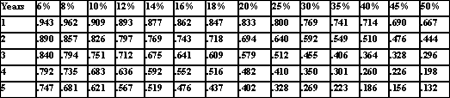# Comparing ROI for multiple business initiatives

## Trying to compare multiple projects requiring different investments? Use this formula from CRM ROI expert Tom Pisello.

I am trying to compare multiple projects requiring different investments. Could you give me a formula for calculating ROI? Here's what I have as inputs:

* Five-year forecast (units)
* Unit price (\$)
* Unit cost (\$)
* Investment (\$)

Do I need any other inputs for calculation?

The ROI calculation is performed by comparing the ratio of net benefits (total rewards – investments) from a project versus the total investment over a set time period – usually between three to five years.

1. First you need to calculate the total sales revenue from this product line over the five year period as:
a. Total Sales Revenue = Total sales units over five years * unit price

2. Now you need to calculate the cost of goods sold from the sales over the five year period:
a. Cost of Goods Sold = Total sales units over five years * unit price

3. Now calculate the net incremental margin contribution from the sale of the product as:
a. Net incremental margin = Total Sales Revenue -- Cost of Goods Sold

4. Finally, to calculate the ROI, you need to factor in the investment:
a. ROI = (Net incremental margin – investment) / investment

A twist to the equation would be to include net present value in the calculations – discounting and adjusting the analysis so that all figures are normalized into today's dollar terms. As money has a time value, in order to have future benefits offset up-front investments, these investments typically have to be much larger than the initial investment – to make up for the lost opportunity from investing the funds elsewhere, and also to make up for inflation. If the project has an up-front investment is large and if the rewards occur greatly in later years, it is particularly important to use net present value variation on ROI (we call it risk-adjusted ROI).

To use net present value (NPV), first you need to have an overall discount rate (minimally the cost of capital for the organization) typically from 8-15%. Once this is known, you analyze the net cash flows look like year over year, applying a net present value discount in each year to translate all cash flows into today dollar terms.

In order to factor NPV in, for each calculation above, you need to analyze the benefits and costs over each year. The formula would look like the following, assuming that the investment made is all initial investment:

((Y1 net incremental margin * Y1 discount rate factor +
Y2 net incremental * Y2 discount rate factor +
Y3 net incremental * Y3 discount rate factor +
Y4 net incremental * Y4 discount rate factor +
Y5 net incremental * Y5 discount rate factor) -
Initial investment ) Divided by the Initial investment

Where the discount rate factor is used per year:Close